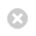Close

Mathematics

Home# Geometry and Topology

Our research interests range from low-dimensional topology and geometric group theory, through algebraic geometry to symplectic geometry, gauge theory, differential geometry and geometric analysis.

The UCL Geometry and Topology Group is part of the UCL Mathematics Department. We have eight faculty members, three postdocs and 14 PhD students. Our research interests include differential geometry and geometric analysis, symplectic geometry, gauge theory, low-dimensional topology and geometric group theory. We are involved with the London School of Geometry and Number Theory (LSGNT), a graduate programme spanning University College London, King's College London and Imperial College London.

Costante Bellettini works in geometric analysis, with special emphasis on regularity questions arising in the calculus of variations and in calibrated geometry, often using methods from geometric measure theory and partial differential equations. He is particularly interested in the impact of such regularity results on questions arising in differential geometry.

Dario Beraldo works in geometric representation theory, especially on the geometric Langlands program. His other interests include derived algebraic geometry, higher category theory, connections to number theory and to mathematical physics.

Aleksander Doan works in differential geometry and symplectic topology. He is particularly interested in the partial differential equations of gauge theory and their relation to enumerative invariants of manifolds with special holonomy, such as Calabi-Yau manifolds.

Lorenzo Foscolo works in differential geometry. His current research interests focus on manifolds with special and exceptional holonomy, in particular G2 and hyperkähler metrics, and gauge theory, and are often directly inspired by theoretical physics.

Jeffrey Galkowski works in microlocal analysis with an emphasis on high frequency spectral and scattering theory problems motivated by mathematical physics. Typically, these problems are posed on non-trivial manifolds where the underlying geometry plays a crucial role.

Selim Ghazouani’s work is about deformation spaces of geometric structures on manifolds. More specifically, I have worked on moduli spaces of structures on surfaces, complex hyperbolic geometry and Lorentzian geometry. I also try to apply geometric techniques to rigidity questions in dynamical systems.

Francis Johnson: Topology of manifolds; low-dimensional topology; the D(2) problem, more generally problems involving the fundamental group; Lie groups and their discrete subgroups; homological algebra; geometric invariant theory.

Mikhail Karpukhin works in geometric analysis. He is primarily interested in harmonic maps, minimal surfaces and their connection to optimisation problems for eigenvalues of elliptic operators.

Lars Louder works in geometric group theory and low dimensional topology, primarily free groups and limit groups.

Yusra Naqvi works in geometric group theory and is especially interested in Coxeter groups, Chevalley groups and buildings. She also works in algebraic combinatorics.

Ruth Reynolds works in noncommutative ring theory, algebraic geometry, and their interactions. Recently her interests include the behaviour of idealizers, a subring of a noncommutative ring, which often exhibit interesting and pathological behaviour.

Ed Segal works in algebraic geometry and homological algebra, with a lot of inspiration from theoretical physics. His interests include derived categories, matrix factorizations, non-commutative resolutions, and topological field theories (mainly the B-model).

Michael Singer has various research interests in differential geometry.  He is currently working on projects in Kaehler geometry, hyperKaehler and ant-self-dual Einstein metrics in four dimensions, and moduli spaces arising in mathematical physics (in particular euclidean monopoles).

Postdoctoral Research Associates

Stephane Geudens is an IMSS fellow working in Poisson geometry and related areas. His current research deals with deformation problems and rigidity phenomena in this context.

Marcello Malagutti works in Harmonic analysis, geometrical analysis of PDEs, microlocal analysis, spectral analysis and scattering theory as well as quantum field theory and quantum mechanics.

Federico Trinca works in differential geometry and geometric analysis including minimal submanifolds, calibrated geometry and manifolds of special holonomy.

Ruoyu Wang works in analysis and PDE on manifolds where global dyanimcs of geodesics quantitatively determine analytic properties of solutions.